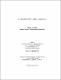## On the solutions of certain congruences2017
##### Authors
Siavashi, Sahar
University of Lethbridge. Faculty of Arts and Science
##### Publisher
Lethbridge, Alta : University of Lethbridge, Dept. of Mathematics and Computer Science
##### Abstract
We study the solutions of certain congruences in different rings. The congruences include a^p-1 ≡ 1 (mod p^2); for integer a > 1 and prime p with p does not divide by a, and a^φ(m) ≡ 1 (mod m^2), for integer m with (a;m) = 1; where j is Euler’s totient function. The solutions of these congruences lead to Wieferich primes and Wieferich numbers. In another direction this thesis explores the extensions of these concepts to other number fields such as quadratic fields of class number one. We also study the solutions of the congruence g^m - g^n ≡ 0 (mod f^m - f^n); where m and n are two distinct natural numbers and f and g are two relatively prime polynomials with coefficients in the field of complex numbers.
##### Keywords
class number one , congruences , quadratic fields , Wieferich numbers , Wieferich primes